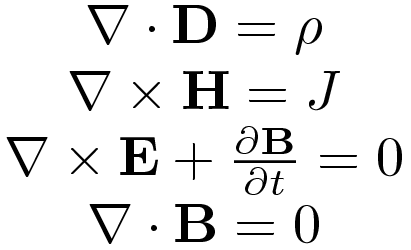#### Jeffro / LatexLambda / 1.1.2### Introduction

Convert Latex notation into Lambda functions in Python. The lambda function is the string value in the func property of the output JSON. The function could be assigned as any ordinary function by evaluating the string using the eval method or by simply copy and pasting the code.

• ##### Input:
• Latex notation string. (Required)

• ##### Output:
• Lambda function as string.

• Parameters to lambda function.

### Examples:

1. Using the summation operator.Input (String):


\sum_{i=1}^{\epsilon}\dfrac{\lambda^{\alpha}}{\beta^{1-\alpha}}


Output (JSON):


{
"func":"lambda α,β,ε,λ: sum([(λ**(α))/(β**(1-α)) for i in range(int(1),int(ε)+1)])",
"params":["α", "β", "ε", "λ"]
}

2. Using the product operator and trigonometric functions.Input (String):


\prod_{i=1}^{n}\frac{\arcsin{\alpha}*\sin{\beta}}{\sqrt{\alpha*\beta}}


Output (JSON):


{
"func":"lambda n,ß,α: np.prod([(np.arcsin(α)*np.sin(ß))/(np.sqrt(α*ß)) for i in range(int(1),int(n)+1)])",
"params":["n", "ß", "α"]
}

3. Evaluating the lambda function.


>>> import numpy as np
>>> func = lambda n,ß,α: np.prod([(np.arcsin(α)*np.sin(ß))/(np.sqrt(α*ß)) for i in range(int(1),int(n)+1))])
>>> func(4,1,1)
3.05236236307


#### Development:

###### TODO Features
• ... Which features would you like to see?

Help support this project. The smallest amount makes a difference.

Contents